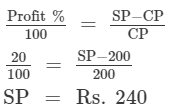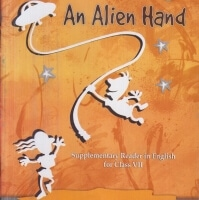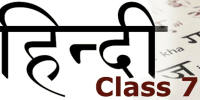Courses

# RD Sharma Solutions - Ex - 12.1, Profit and Loss, Class 7, Math Class 7 Notes | EduRev

## Class 7: RD Sharma Solutions - Ex - 12.1, Profit and Loss, Class 7, Math Class 7 Notes | EduRev

The document RD Sharma Solutions - Ex - 12.1, Profit and Loss, Class 7, Math Class 7 Notes | EduRev is a part of the Class 7 Course RD Sharma Solutions for Class 7 Mathematics.
All you need of Class 7 at this link: Class 7

#### Question 1:

Given the following values, find the unknown values:
(i) C.P. = Rs 1200,      S.P. = Rs 1350,     Profit/Loss = ?
(ii) C.P. = Rs 980,       S.P. = Rs  940,      Profit/Loss = ?
(iii) C.P. = Rs 720,      S.P. = ?,                Profit = Rs 55.50
(iv) C.P. = ?                  S.P. = Rs 1254,     Loss = Rs 32

(i) CP =  Rs.. 1200, SP = Rs.. 1350
CP < SP.  So, profit.
Profit = Rs. (1350 - 1200) = Rs. 150

(ii) CP = Rs. 980, SP = Rs. 940
CP > SP.  So, loss.
Loss  =   Rs. (980 - 940) = Rs. 40

(iii)  CP = Rs. 720, SP = ?, profit = Rs. 55.50
Profit = SP - CP
⇒   Rs. 55.50 = SP - Rs. 720
⇒   SP = Rs. (55.50 + 720) = Rs. 775.50

(iv) CP = ?, SP = Rs. 1254, loss = Rs. 32
⇒ Loss = CP - SP
⇒ Rs. 32 = CP - Rs. 1254
⇒ CP = Rs. (1254 + 32) = Rs. 1286

#### Question 2:

Fill in the blanks in each of the following:
(i) C.P. = Rs 1265,      S.P. = Rs 1253,    Loss = Rs .....
(ii) C.P. = Rs ....,         S.P. = Rs 450,      Profit = Rs 150
(iii) C.P. = Rs 3355,    S.P. = Rs 7355,    .... = Rs ....
(iv) C.P. = Rs ....,        S.P. = Rs 2390,    Loss = Rs 5.50

(i) CP = Rs. 1265, SP = Rs. 1253
Loss = CP - SP = Rs. (1265 - 1253) = Rs. 12

(ii) CP = ?, SP = Rs. 450, profit = Rs. 150
Profit = SP - CP
⇒  Rs. 150 = Rs. 450 - CP
⇒  CP = Rs. (450 - 150) = Rs. 300

(iii) CP = Rs. 3355, SP = Rs. 7355,
Here SP > CP, so profit.
Profit = SP - CP
⇒ Profit = Rs. (7355 - 3355) = Rs. 4000

(iv) CP = ?, SP = Rs. 2390, loss = Rs. 5.50
Loss = CP - SP
⇒ Rs. 5.50 = CP - Rs. 2390
⇒ CP = Rs. (5.50 + 2390) = Rs. 2395.50

#### Question 3:

Calculate the profit or loss and profit or loss per cent in each of the following cases:
(i) C.P. = Rs 4560, S.P. = Rs 5000
(ii) C.P. = Rs 2600, S.P. = Rs 2470
(iii) C.P. = Rs 332, S.P. = Rs 350
(iv) C.P. = Rs 1500, S.P. = Rs 1500

(i) CP = Rs. 4560, SP = Rs. 5000
Here, SP > CP. So, profit.
Profit = SP - CP = Rs. (5000 - 4560)= Rs. 440
Profit % = {(Profit/CP) × 100}% = {(440/4560) × 100}% = {0.0965 × 100}% = 9.65%

(ii) CP = Rs. 2600, SP = Rs. 2470. Here, CP > SP.  So, loss.
Loss = CP - SP = Rs. (2600 - 2470) = Rs. 130
Profit% = {(Profit/CP) × 100}% =  {(130/2600) × 100}% = {0.05 ×  100}% = 5%

(iii) CP = Rs. 332, SP= Rs. 350. Here, SP > CP.  So, profit.
Profit = SP - CP = Rs. (350 - 332) = Rs. 18
Profit% = {(Profit/CP) × 100}%  = {(18/332) × 100}%  = {0.054  × 100}% = 5.4%

(iv) CP = Rs. 1500, SP = Rs. 1500
SP = CP.  So, neither profit nor loss.

#### Question 4:

Find the gain or loss per cent, when:
(i) C.P. = Rs 4000 and gain = Rs 40.
(ii) S.P. = Rs 1272 and loss = Rs 328
(iii) S.P. = Rs 1820 and gain = Rs 420.

(i) CP = Rs. 4000, gain = Rs. 40
Gain % = {(Gain/CP) × 100}% = {(40/4000) × 100}% = (0.01 × 100)% = 1%

(ii) SP = Rs. 1272, loss = Rs. 328
Loss = CP - SP
Hence, CP = Loss+ SP = Rs. 328 + Rs. 1272 = Rs. 1600
Loss % = {(Loss/CP) × 100}% = {(328/1600) × 100%  = 20.5%

(iii) SP = Rs. 1820, gain = Rs. 420
Gain = SP - CP
CP = 1820 - 420 = Rs. 1400
Gain % = {(Gain/CP) × 100}%  = {(420/1400) × 100% = 30%

#### Question 5:

Find the gain or loss per cent, when:
(i) C.P. = Rs 2300, Overhead expenses = Rs 300 and gain = Rs 260.
(ii) C.P. = Rs 3500, Overhead expenses = Rs 150 and loss = Rs 146

(i) CP = Rs. 2300, overhead expenses = Rs. 300, gain = Rs. 260
Gain % =  {(Gain/(CP + overhead expenses)} × 100 = {260/(2300 + 300} × 100 = {260/2600} × 100 = 10%

(ii) CP = Rs. 3500, overhead expenses = Rs. 150, loss = Rs. 146

Loss % = {( Loss/(CP + overhead expenses)} × 100 = {146/(3500+ 150)} × 100
= {146/3650} × 100
= 14600/3650 = 4%

#### Question 6:

A grain merchant sold 600 quintals of rice at a profit of 7%. If a quintal of rice cost him Rs 250 and his total overhead charges for transportation, etc. were Rs 1000 find his total profit and the selling price of 600 quintals of rice.

Cost of 1 quintal of rice = Rs. 250
Cost of 600 quintals of  rice = 600 × 250 =  Rs. 150000
Total CP = Rs. (150000 + 1000) = Rs. 151000
Profit % = (Profit/CP) × 100
7 = (P/151000) × 100
P = 1510 × 7 = Rs. 10570
Profit = Rs. 10570
SP = CP + profit = Rs. (151000 + 10570) = Rs. 161570

#### Question 7:

Naresh bought 4 dozen pencils at Rs 10.80 a dozen and sold them for 80 paise each. Find his gain or loss percent.

Cost of 1 dozen pencils = Rs. 10.80
Cost of 4 dozen pencils = 4 × 10.80 =  Rs. 43.2

Selling price of each pencil = 80 paise
Total number of pencils = 12 × 4 = 48
SP of 48 pencils = 48 × 80 paise = 3840 paise = Rs. 38.40

Here, SP < CP.
Loss = CP - SP = Rs. (43.2 - 38.4) = Rs. 4.8
Loss % = (Loss/CP) × 100 = (4.8/43.2) × 100 = 480/43.2 = 11.11%

#### Question 8:

A vendor buys oranges at Rs 26 per dozen and sells them at 5 for Rs 13. Find his gain per cent.

CP of 1 dozen oranges = Rs. 26
CP of 1 orange = 26/12 = Rs. 2.16
CP of 5 oranges = 2.16 × 5 = Rs. 10.8

Now, SP of  5 oranges = Rs. 13

Gain = SP - CP = Rs. (13 - 10.8) = Rs. 2.2
Gain % = (Gain/CP) × 100 = (2.2/10.8) × 100 = 20.3%

#### Question 9:

Mr Virmani purchased a house for Rs 365000 and spent Rs 135000 on its repairs. If he sold it for Rs 550000, find his gain percent.

Amount Mr. Virmani paid to purchase the house = Rs. 365000
Amount he spent on repair = Rs. 135000
Total amount he spent on the house (CP) = Rs. (365000 + 135000) = Rs. 500000
SP of the house = Rs. 550000
Gain = SP - CP = Rs. (550000 - 500000) = Rs. 50000

Gain % = (Gain/CP) ×  100 = (50000/500000) × 100 = 5000000/500000 = 10%

#### Question 10:

Shikha purchased a wrist watch for Rs 840 and sold it to her friend Vidhi for Rs 910. Find her gain percent.

The cost price of the wristwatch that Shikha purchased, CP = Rs. 840
The price at which she sold it, SP = Rs. 910
Gain = SP - CP
= (910 - 840) = Rs. 70

Gain % = (Gain/CP) ×  100 = (70/840) × 100 = 7000/840 = 8.3%

#### Question 11:

A business man makes a 10% profit by selling a toy costing him Rs 120. What is the selling price?

CP = Rs. 12
Profit % = 10
We now that

SP = {(100 + profit %)/100} × CP
= {(100+ 10)/100} ×  120
= {(110/100)} × 120 = 1.1 × 120 = Rs. 132

#### Question 12:

Harish purchased 50 dozen bananas for Rs 135. Five dozen bananas could not be sold because they were rotten. At what price per dozen should Harish sell the remaining bananas so that he makes a profit of 20%?

Cost price of 50 dozens bananas that Harish purchased, CP = Rs. 135

Bananas left after removing 5 dozen rotten bananas = 45 dozens

Effective CP of one dozen bananas = Rs. 135/45 = Rs. 3

Calculating the price at which Harish should sell each dozen bananas to make a profit of 20% (or 1/5), we get
Profit = Gain/CP =  (SP - CP)/CP
15 = SP 33SP = Rs. 3.6015 = SP- 33SP = Rs. 3.60

Harish should sell the bananas at Rs. 3.60 a dozen in order to make a profit of 20%.

#### Question 13:

A woman bought 50 dozen eggs at Rs 6.40 a dozen. Out of these 20 eggs were found to be broken. She sold the remaining eggs at 55 paise per egg. Find her gain or loss percent.

Cost of one dozen eggs = Rs. 6.40
Cost of 50 dozen eggs = 50 × 6.40 = Rs. 320

Total number of eggs = 50 × 12 = 600
Number of eggs left after removing the broken ones = 600 - 20 = 580

SP of 1 egg = 55 paise
So, SP  of 580 eggs = 580 × 55 = 31900 paise = Rs. 31900/100 = Rs. 319

Loss = CP - SP = Rs. (320-319) = Re. 1
Loss % = (Loss/CP) × 100 = (1/320) × 100 = 0.31%

#### Question 14:

Jyotsana bought 400 eggs at Rs 8.40 a dozen. At what price per hundred must she sell them so as to earn a profit of 15%?

Cost of eggs per dozen = Rs. 8.40
Cost of 1 egg = 8.40/12 = Rs. 0.7
Cost of 400 eggs = 400 × 0.7 = Rs. 280
Calculating the price at which Jyotsana should sell the eggs to earn a profit of 15%, we get
15% of 280 + 280
=  {(15/100) × 280} + 280 = {4200/100} + 280 = 42 + 280 = Rs. 322

So, Jyotsana must sell the 400 eggs for Rs. 322 in order to earn a profit of 15%.
Therefore, the SP per one hundred eggs = Rs. 322/4 = Rs. 80.50.

#### Question 15:

A shopkeeper makes a profit of 15% by selling a book for Rs 230. What is the C.P. and the actual profit?

Given that the SP of a book = Rs. 230
Profit % = 15
Since
CP = (SP × 100) ÷ (100 + profit %)
CP = (230× 100)  ÷ (100 + 15)
CP = 23000 ÷ 115 = Rs. 200
Also,
Profit = SP - CP = Rs. (230 - 200) = Rs. 30
Actual profit = Rs. 30

#### Question 16:

A bookseller sells all his books at a profit of 10%. If he buys a book from the distributor at Rs 200, how much does he sell it for?

Given
Profit % = 10%
CP = Rs. 200
Since
SP = {(100 + profit %)/100} × CP
= {(100 + 10)/100} × 200
= {110/100} × 200
= Rs. 220

The bookseller sells the book for Rs. 220.

#### Question 17:

A flowerist buys 100 dozen roses at Rs 2 a dozen. By the time the flowers are delivered, 20 dozen roses are multilated and are thrown away. At what price should he sell the rest if he needs to make a 20% profit on his purchase?

Cost of 1 dozen roses = Rs. 2
Number of roses bought by the florist = 100 dozens
Thus, cost price of 100 dozen roses = 2  × 100 =  Rs. 200

Roses left after discarding the mutilated ones = 80 dozens

Calculating the price at which the florist should sell the 80 dozen roses in order to make a profit of 20%, we haveTherefore, the SP of the roses should be Rs. 240/80 = Rs. 3 per dozen.

#### Question 18:

By selling an article for Rs 240, a man makes a profit of 20%. What is his C.P.? What would his profit percent be if he sold the article for Rs 275?

Let CP = Rs. x
SP = Rs. 240
Let profit be Rs. P.

Now, profit % = 20%
Since
Profit % = (Profit/CP) × 100
⇒ 20 = (P/x) × 100
⇒ P = 20x/100 = x/5

Profit = SP - CP = 240 - x
⇒ P = 240 -
⇒ x/5 = 240 - x
⇒ 240 =  x + x/5
⇒ 240 = 6x/5
x = 1200/6 = 200
So, CP = Rs. 200

New SP = Rs. 275 and CP = Rs. 200
Profit % = {(SP - CP)/CP} × 100 = {(275 - 200)/200} × 100 = (75/200) × 100
= 7500/200 = 37.5%

The document RD Sharma Solutions - Ex - 12.1, Profit and Loss, Class 7, Math Class 7 Notes | EduRev is a part of the Class 7 Course RD Sharma Solutions for Class 7 Mathematics.
All you need of Class 7 at this link: Class 7Use Code STAYHOME200 and get INR 200 additional OFF Use Coupon Code
All Tests, Videos & Notes of Class 7: Class 7

### Top Courses for Class 7## RD Sharma Solutions for Class 7 Mathematics

97 docs

### Top Courses for Class 7Track your progress, build streaks, highlight & save important lessons and more!

,

,

,

,

,

,

,

,

,

,

,

,

,

,

,

,

,

,

,

,

,

,

,

,

,

,

,

,

,

,

;